# ISEE Middle Level Math : Numbers and Operations

## Example Questions

← Previous 1 3 4 5 6 7 8 9 93 94

### Example Question #1 : Numbers And Operations

What is the least common multiple of 5, 12, and 15?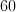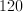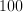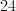Explanation:

You can write out the multiples of each number, and find the first number that is common to all of them.

5: 5, 10, 15, 20, 25, 30, 35, 40, 45, 50, 55, 60

12: 12, 24, 36, 48, 60

15: 15, 30, 45, 60

### Example Question #1 : Ratio And Proportion

What is the greatest common factor of 12, 24 and 96?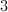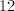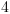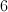Explanation:

To find the greatest common factor of three numbers, first factor each number as a product of prime numbers: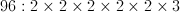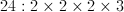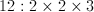Now take all of the factors that are in common between 12, 24 and 96.  In this case, that is 2, 2 and 3.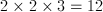### Example Question #1 : Ratio And Proportion

Logan needs to answer 60 questions to finish his test. If he has answered 25% of the questions in 20 minutes, how long will it take him to complete the entire test?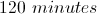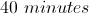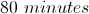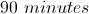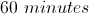Explanation:

To solve:

First, find the number of answers completed by calculating: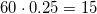Then, find how long it would take him to complete the 60 question test if for every 20 minutes he answer 15 questions: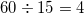(sets of 15 questions)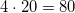minutes altogether to finish the test.

### Example Question #1 : How To Find A Ratio

Which ratio is equivalent to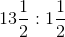?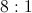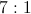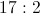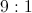Explanation:

A ratio can be rewritten as a quotient; do this, and simplify it.Rewrite as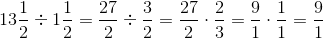or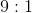### Example Question #1 : Numbers And Operations

I have 5 white chocolates, 3 milk chocolates, and 9 dark chocolates. What is the ratio of white chocolates to the other types of chocolates?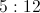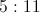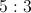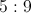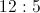Explanation:

There are 5 white chocolates. There are a total of 12 milk and dark chocolates. The ratio of white to other chocolates is 5:12.

### Example Question #1 : Ratio And Proportion

In February, there were 12 rainy days, 6 cloudy days and 10 sunny days. What was the ratio of sunny days in February?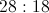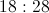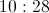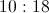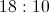Explanation:

Identify the number of sunny days in February :Then, creating a ratio,  show the number of sunny days compared to the total number of days in the month of February (28):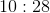Answer:### Example Question #891 : Isee Middle Level (Grades 7 8) Mathematics Achievement

Express the following ratio in simplest form: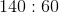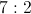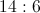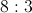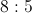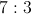Explanation:

Rewrite this in fraction form for the sake of simplicity, and divide both numbers by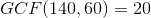: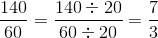The ratio, simplified, is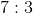.

### Example Question #3 : How To Find A Ratio

Express this ratio in simplest form: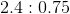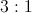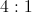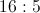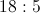Explanation:

A ratio involving decimals can be simplified as follows:

First, move the decimal point over a common number of places in each number so that both numbers become whole. In this case, it would be two places: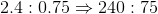(note the addition of a zero at the end of the first number)

Now, rewrite as a fraction and divide both numbers by: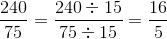The ratio, simplified, is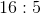### Example Question #2 : Numbers And Operations

Express this ratio in simplest form: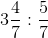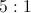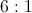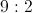Explanation:

A ratio of fractions can best be solved by dividing the first number by the second. Rewrite the mixed fraction as an improper fraction, rewrite the problem as a multiplication by taking the reciprocal of the second fraction, and cross-cancel: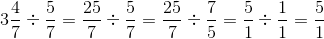The ratio, simplified, is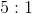.

### Example Question #1 : Numbers And Operations

Write as a unit rate: 450 words typed in 25 minutes.

17 words per minute

19 words per minute

18 words per minute

22 words per minute

23 words per minute

18 words per minute

Explanation:

Divide the number of words by the number of minutes to get words per minute: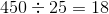words per minute

← Previous 1 3 4 5 6 7 8 9 93 94

### All ISEE Middle Level Math Resources